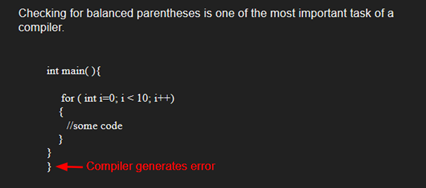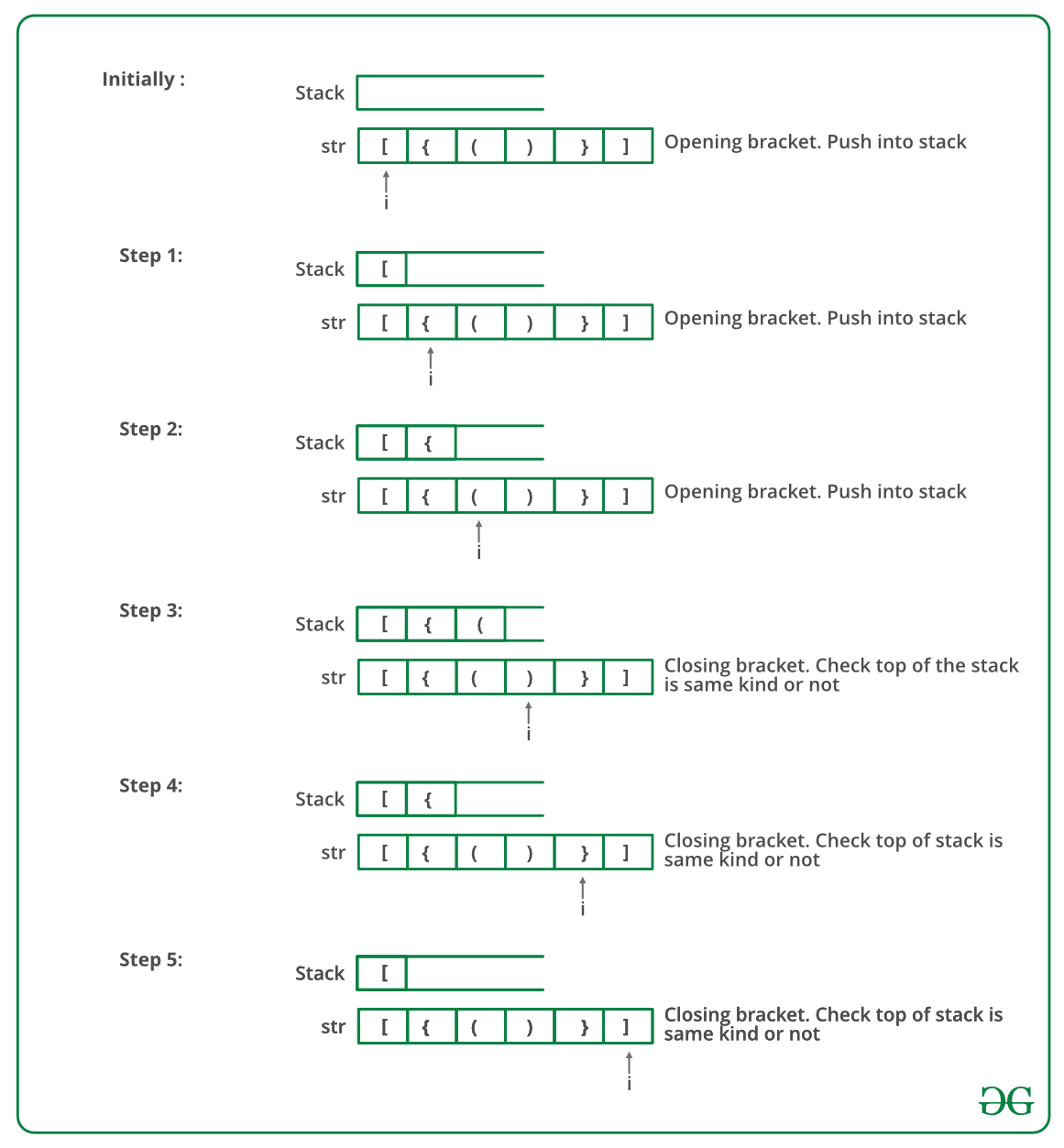GFG App
Open AppBrowser
Continue

# Java Program To Check For Balanced Brackets In An Expression (Well-Formedness) Using Stack

Given an expression string exp, write a program to examine whether the pairs and the orders of “{“, “}”, “(“, “)”, “[“, “]” are correct in exp.

Example

Input: exp = “[()]{}{[()()]()}”
Output: Balanced

Input: exp = “[(])”
Output: Not BalancedAlgorithm:

• Declare a character stack S.
• Now traverse the expression string exp.
1. If the current character is a starting bracket (‘(‘ or ‘{‘ or ‘[‘) then push it to stack.
2. If the current character is a closing bracket (‘)’ or ‘}’ or ‘]’) then pop from stack and if the popped character is the matching starting bracket then fine else brackets are not balanced.
• After complete traversal, if there is some starting bracket left in stack then “not balanced”

Below image is a dry run of the above approach:Below is the implementation of the above approach:

## Java

 `// Java program for checking ` `// balanced brackets ` `import` `java.util.*; ` ` `  `public` `class` `BalancedBrackets { ` ` `  `    ``// function to check if brackets are balanced ` `    ``static` `boolean` `areBracketsBalanced(String expr) ` `    ``{ ` `        ``// Using ArrayDeque is faster than using Stack class ` `        ``Deque stack ` `            ``= ``new` `ArrayDeque(); ` ` `  `        ``// Traversing the Expression ` `        ``for` `(``int` `i = ``0``; i < expr.length(); i++)  ` `        ``{ ` `            ``char` `x = expr.charAt(i); ` ` `  `            ``if` `(x == ``'('` `|| x == ``'['` `|| x == ``'{'``)  ` `            ``{ ` `                ``// Push the element in the stack ` `                ``stack.push(x); ` `                ``continue``; ` `            ``} ` ` `  `            ``// If current character is not opening ` `            ``// bracket, then it must be closing. So stack ` `            ``// cannot be empty at this point. ` `            ``if` `(stack.isEmpty()) ` `                ``return` `false``; ` `            ``char` `check; ` `            ``switch` `(x) { ` `            ``case` `')'``: ` `                ``check = stack.pop(); ` `                ``if` `(check == ``'{'` `|| check == ``'['``) ` `                    ``return` `false``; ` `                ``break``; ` ` `  `            ``case` `'}'``: ` `                ``check = stack.pop(); ` `                ``if` `(check == ``'('` `|| check == ``'['``) ` `                    ``return` `false``; ` `                ``break``; ` ` `  `            ``case` `']'``: ` `                ``check = stack.pop(); ` `                ``if` `(check == ``'('` `|| check == ``'{'``) ` `                    ``return` `false``; ` `                ``break``; ` `            ``} ` `        ``} ` ` `  `        ``// Check Empty Stack ` `        ``return` `(stack.isEmpty()); ` `    ``} ` ` `  `    ``// Driver code ` `    ``public` `static` `void` `main(String[] args) ` `    ``{ ` `        ``String expr = ``"([{}])"``; ` ` `  `        ``// Function call ` `        ``if` `(areBracketsBalanced(expr)) ` `            ``System.out.println(``"Balanced "``); ` `        ``else` `            ``System.out.println(``"Not Balanced "``); ` `    ``} ` `} `

Output

`Balanced`

Time Complexity: O(n)
Auxiliary Space: O(n) for stack.

Please refer complete article on Check for Balanced Brackets in an expression (well-formedness) using Stack for more details!

My Personal Notes arrow_drop_up